On the meadow grazing horses, cows and sheep, together less than 200. If cows were 45 times more, horses 60 times more and sheep 35 times more than there are now, their numbers would equall. How many
horses, cows and sheep are on the meadow together?

Result

x1 =  85
x2 =  170

Solution:

x = a+b+c < 200
b = 45/60 a
c = 45/35 a
a + 45/60 a + 45/35 a < 200
LCD(11, 4560, 4535) = LCM(1, 60, 35) = 22×3×5×7 = 420
11 = 420420
4560 = 315420
4535 = 540420

420 k + 315 k + 540 k < 84000
1275 k < 84000
17 k < 1120

x = 56+42+72 = 170
x = 28+21+36 = 85

Leave us a comment of example and its solution (i.e. if it is still somewhat unclear...):Be the first to comment!To solve this example are needed these knowledge from mathematics:

Do you want to calculate least common multiple two or more numbers? Need help calculate sum, simplify or multiply fractions? Try our fraction calculator.

Next similar examples:

1. Sheep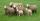Shepherd tending the sheep. Tourists asked him how much they have. The shepherd said, "there are fewer than 500. If I them lined up in 4-row 3 remain. If in 5-row 4 remain. If in 6-row 5 remain. But I can form 7-row." How many sheep have herdsman?
2. 9.A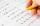9.A to attend more than 20 students but fewer than 40 students. A third of the pupils wrote a math test to mark 1, the sixth to mark 2, the ninth to mark 3. No one gets mark 4. How many students of class 9.A wrote a test to mark 5?
3. Snowman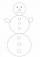In a circle with a diameter 50 cm are drawn 3 circles /as a snowman/ where: its diameters are integers, each larger circle diameter is 3 cm larger than the diameter of the previous circle. Determine snowman height if we wish highest snowman.
4. Logistics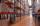Head of logistics department informed the meeting of management of the same item is currently not available in sufficient quantity in all three warehouses of the company. We have the total 135 pieces. It was 37 pieces more in the warehouse No. 3 than in th
5. Classroom 4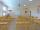In a class of 36 pupils, 2/3 are girls. How much it is in a class girls and boys?
6. Theorem proveWe want to prove the sentence: If the natural number n is divisible by six, then n is divisible by three. From what assumption we started?
7. Tunnels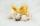Mice had built an underground house consisting of chambers and tunnels: • each tunnel leading from the chamber to the chamber (none is blind) • from each chamber lead just three tunnels into three distinct chambers, • from each chamber mice can get to any
8. Amazing numberAn amazing number is name for such even number, the decomposition product of prime numbers has exactly three not necessarily different factors and the sum of all its divisors is equal to twice that number. Find all amazing numbers.
9. Unknown numberUnknown number is divisible by exactly three different primes. When we compare these primes in ascending order, the following applies: • Difference first and second prime number is half the difference between the third and second prime numbers. • The produ
10. Nuts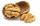How many we must have at least nuts if we can equally divide it to 10 children, 11 children or 19 children and any nut left?
11. Numbers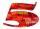Write smallest three-digit number, which in division 5 and 7 gives the rest 2.
12. Pyramid Z8–I–6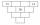Each brick of pyramid contains one number. Whenever possible, the number in each brick is lowest common multiple of two numbers of bricks lying directly above it. That number may be in the lowest brick? Determine all possibilities.
13. Four classses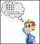Students of all 7, 8 and 9 classes in one school may take up 4,5,6 and 7 abreast and nobody will left. How many is the average count of pupils in one class if there are always four classes each grade?
14. Seamstress 2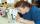Seamstress bought two kinds of textile in whole meters. One at 50 SKK and the second 70 SKK per meter. How many meter bought from this two textiles when paid totally 1540 SKK?
15. DivisorsThe sum of all divisors unknown odd number is 2112. Determine sum of all divisors of number which is twice of unknown numbers.
16. Odd/even numberPick any number. If that number is even, divide it by 2. If it's odd, multiply it by 3 and add 1. Now repeat the process with your new number. If you keep going, you'll eventually end up at 1. Every time. Prove. ..
17. Two months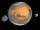Mars has two months, Phobos and Deimos. Phobos orbits around Mars in 7 hours 39 minutes and Deimos in 30 h 14 min. How long will repeat the relative position of the three celestial bodies?